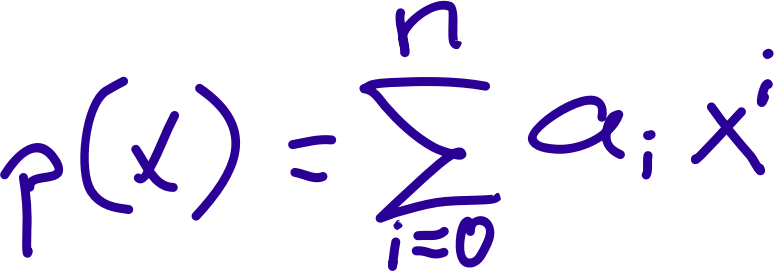## Polynomial Definition

The definition of a polynomial in summation notation, hand-written by yours truly (at age 67). The 'x' is the argument of the function p(x), the 'a' is an array of n+1 parameters (called coefficients). For each 'i' (integers ranging from 0 to n, all inclusive), the corresponding coefficient a[i] is multiplied by the i-th power of the variable x, and the results of these multiplications are all added up.

For the programmers among us, this would be written in the C programming language as:

for (i = 0, p = 0.0; i

Tags
Safe for Work?
Yes